# Explanation of results

## Electrostatic energy

The density of the electrostatic energy in a point is expressed by means of the electric field quantities D and E by the relation: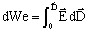which can also be written for a linear and isotropic medium as: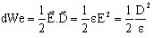The electrostatic energy in a volume region is equal to: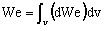## Electrostatic force and torque

The electrostatic forces and torques are computed by the virtual work method.

The corresponding region should be surrounded by air.

## Electric charge on the surface of a conductor

The charge carried by a surface of a conductor is equal to: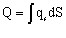This computation can be carried out on an equipotential surface, i.e.:

• on the surface of a perfect conductor
• on the surface of imposed potential type (with uniform V0)

## Capacitance computation

It is possible to compute the capacitance between two conductors in two different ways:

• by using the expression of electrostatic energy (We):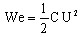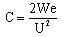• by using the expression of electric charge (Q):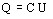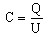where U represents the potential difference between the conductors.

The second method is more interesting in term of computing time for computation of a matrix of capacitance.